# Civil Engineering - Theory of Structures

### Exercise :: Theory of Structures - Section 3

16.

The forces acting on the bar as shown in the given figure introduce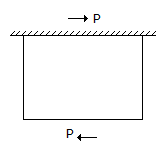A. compressive stress B. tensile stress C. shear stress D. none of these.

Explanation:

No answer description available for this question. Let us discuss.

17.

The equation of a parabolic arch of span l and rise h, is given by

 A.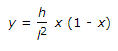B.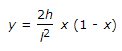C.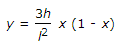D.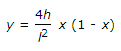Explanation:

No answer description available for this question. Let us discuss.

18.

Pick up the correct statement from the following:

 A. A wire wound in spiral form, is called a helical spring B. The pitch of a close coil spring, is very small C. The angle made by the coil with horizontal, is called the angle of helix D. In the open coil helical spring, the angle of helix is comparatively large E. All the above.

Explanation:

No answer description available for this question. Let us discuss.

19.

The horizontal thrust on the ends of a two hinged semicircular arch of radius R carrying

 A. a uniformly distributed load ω per unit run over its right half span, is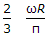B. a uniformly distributed load ω per unit run over its entire span is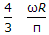C. a distributed load varying from zero at the left end to ω per unit horizontal run at the right end, isD. all the above.

Explanation:

No answer description available for this question. Let us discuss.

20.

Maximum principal stress theory for the failure of a material at elastic point, is known

 A. Guest's or Trecas' theory B. St. Venant's theory C. Rankine's theory D. Haig's theory E. Von Mises's theory.